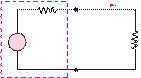### Create an Account

Already have account?

### Forgot Your Password ?

Home / Questions / A 220 V electric heater has two heating coils which can be switched such that either coil...

# A 220 V electric heater has two heating coils which can be switched such that either coil can be used independently or the two can be connected in series or parallel yielding a total of four

A 220-V electric heater has two heating coils which can be switched such that either coil can be used independently or the two can be connected in series or parallel, yielding a total of four possible configurations. If the warmest setting corresponds to 2,000-W power dissipation and the coolest corresponds to 300 W, find

a.    The resistance of each of the two coils.

b.    The power dissipation for each of the other two possible arrangements.

#### Sections 2.5, 2.6: Circuit Elements and their i-v Characteristics, Resistance and Ohm’s Law

2.33      For the circuit shown in Figure P2.33, determine the power absorbed by the 5-resistor.

ratio of load power to source power.

VS  = 12 V         RS  = 5 kQ          RL  = 7 kQIT

 RS

 +

+

 _

 V

 S

VT                   RL

_

Nonideal source

Jun 19 2020 View more View Less

#### Answer (Solved)Subscribe To Get Solution